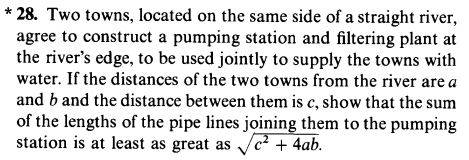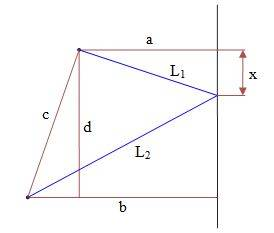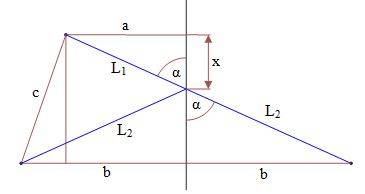# Min max problem: length of pipe from 2 towns to the river

• Karol

## Homework Statement## Homework Equations

Minimum/Maximum occurs when the first derivative=0

## The Attempt at a Solution$$l_1^2+l_2^2=(a^2+x^2)+[b^2+(d-x)^2]=a^2+b^2+x^2+\left[ \sqrt{c^2-(b-a)^2}-x \right]^2$$
$$(l_1^2+l_2^2)'=2x+2\left[ \sqrt{c^2-(b-a)^2}-x \right](-1)$$
$$(l_1^2+l_2^2)'=0~~\rightarrow~~x=\frac{1}{2}\sqrt{c^2-(b-a)^2}=\frac{d}{2}$$
The minimum (or maximum?) distance is when the station is in the middle of the vertical distance between the cities
The total pipe's distance is:
$$Dist=\sqrt{l_1}+\sqrt{l_2}=\sqrt{a^2+x^2}+\sqrt{b^2+x^2}=\sqrt{a^2+\frac{d^2}{4}}+\sqrt{b^2+\frac{d^2}{4}}$$
It doesn't give ##~\sqrt{c^2-4ab}##

#### Attachments

You are supposed to minimise the sum of the lengths (i.e., ##L_1 + L_2##) not the sum of the squares of the lengths (##L_1^2 + L_2^2##).

Hint: Imagine b is on the other side but d is still the same.

You are supposed to minimise the sum of the lengths (i.e., ##L_1 + L_2##) not the sum of the squares of the lengths (##L_1^2 + L_2^2##).
But if the square of the lenghts is minimum then the length is also minimum
I will think about the hint that b is on the other side

But if the square of the lenghts is minimum then the length is also minimum
No, this is not true. It is true for a single length, not for the sum of lengths.

To expand a bit on that. Note that
$$(L^2)' = 2LL'$$
so, for a single length, ##L' = 0## if ##(L^2)' = 0##. However, you have the sum of two lengths and
$$((L_1+L_2)^2)' = 2(L_1+L_2) (L_1+L_2)' = [L_1^2 + L_2^2 + 2L_1 L_2]' = (L_1^2 + L_2^2)' + 2(L_1 L_2' + L_1' L_2).$$
Note that ##(L_1^2 + L_2^2)' = 0## no longer guarantees that ##(L_1 + L_2)' = 0## as there are additional terms on the right-hand side.

Hint: Imagine b is on the other side but d is still the same.$$\frac{a}{x}=\frac{b}{\sqrt{c^2-(b-a)^2}-x}~\rightarrow~x=\frac{a\sqrt{c^2-(b-a)^2}}{a+b}$$
$$l_1+l_2=...=\sqrt{c^2+4ab}$$

#### Attachments

View attachment 229938
$$\frac{a}{x}=\frac{b}{\sqrt{c^2-(b-a)^2}-x}~\rightarrow~x=\frac{a\sqrt{c^2-(b-a)^2}}{a+b}$$
$$l_1+l_2=...=\sqrt{c^2+4ab}$$

You are given a,b,c. Your first task is to find d. Then find L1+L2 when it is the shortest configuration.

You are given a,b,c. Your first task is to find d. Then find L1+L2 when it is the shortest configuration.
I think the ##\ldots = \sqrt{c^2 + 4ab}## means he solved it ...